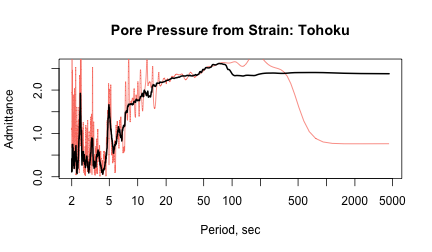Here I show how the modeling tools in kitagawa can be used to study actual data. Specifically, I will show records of strain and pore-pressure from borehole stations in the Plate Boundary Observatory in a manner similar to the approaches taken in @barbour2011 and @barbour2015.

library(kitagawa)

## Loaded kitagawa (2.2.2) -- Spectral response of water wells


## Pore Pressure Changes from Teleseismic Strains

We first load the psd package, which includes a suitable dataset for this example. In particular, we're interested in assessing the frequency-dependent relationship between pore pressure $$p$$ and areal strain $$E_A$$[Relative changes in borehole diameter, which can be related to volume strain in the rock] during the seismic portion of the record.

library(psd)

## Loaded psd (1.0.1) -- Adaptive multitaper spectrum estimation

data(Tohoku)
toh_orig <- with(subset(Tohoku, epoch=='seismic'), {
cbind(
scale(1e3*areal, scale=FALSE), # scale strain to nanostrain, remove mean
scale(1e2*pressure.pore, scale=FALSE) # scale hPa to Pa, remove mean
)
})
colnames(toh_orig) <- c('input','output')
toh.dat <- window(ts(toh_orig), 100, 2400)


Note how the records of this earthquake – the 2011 $$M_W 9$$ Tohoku-Oki earthquake some thousands of kilometers away – are very nearly a mirror image of each other: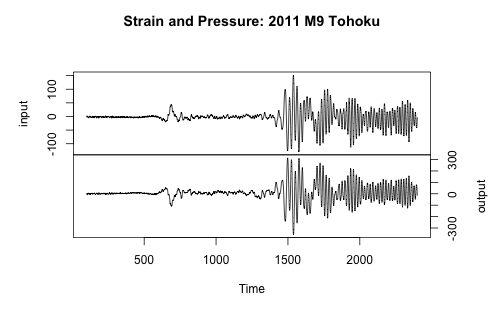This indicates that the pore pressure response can be modeled as a convolution of an input signal (dynamic strain) and transfer function ($$p = G \star E_A$$). It also says that energy carried by the seismic wavetrain is focused predominately at long periods and very nearly harmonic; this is consistent with the theory of linear poroelasticity, which predicts that $p \approx - \frac{4}{3} B \mu E_A$ assuming an undrained Poisson's ratio of $$1/3$$, where $$B$$ is the Skempton's coefficient and $$\mu$$ is the elastic shear modulus of the fluid-saturated rock. In this case the (scalar) proportionality implied by the timeseries is -2.265 $$GPa / \epsilon$$, but we will see how this is actually frequency dependent.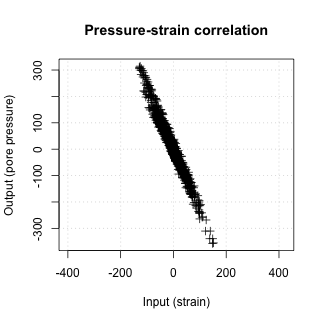## Cross-Spectrum Estimation

First let's use Don Percival's package sapa to estimate a cross spectrum between pressure and strain, treating strain as the input to the system and pressure as the output:

library(sapa)
k <- 2*130
toh.cs <- sapa::SDF(toh.dat, method='multitaper', n.taper=k, sampling.interval=1)
print(toh.cs)

## Cross-Spectral Density Function estimation for toh.dat
## ------------------------------------------------------
## Cross-SDF labels          : S11 S12 S22
## Length of series          : 2301
## Sampling interval         : 1
## Frequency resolution (Hz) : 0.0002172968
## Centered                  : TRUE
## Recentered                : FALSE
## Single-sided              : TRUE
## Method                    : Multitaper
## Number of tapers          : 260
## Taper: sine
##    Number of points: 2301
##    Number of tapers: 260
##    Normalized: TRUE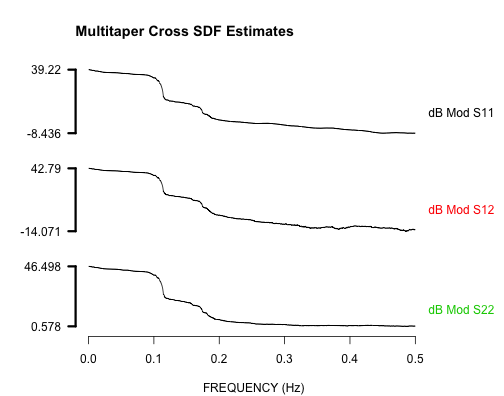### Estimating the Response: Coherence, Admittance, and Phase

If the results of the SDF computation give a matrix of complex spectra $$[S_{11}, S_{12}, S_{22}]$$, the coherence spectrum $$\gamma^2$$ can be calculated by $\gamma^2 = \frac{\left|S_{12}\right|^2}{S_{11} S_{22}},$ the admittance spectrum (or gain) $$G$$ can be calculated from $G = \gamma \sqrt{S_{22} / S_{11}},$ and the phase spectrum $$\Phi$$ can be calculated from $\Phi = \arg{S_{12}}$

f <- as.vector(attr(toh.cs, 'frequency'))
lf <- log10(f)
p <- 1/f
lp <- log10(p)
S <- as.matrix(toh.cs)
colnames(S) <- attr(toh.cs, 'labels')
S11 <- S[,'S11']
S12 <- S[,'S12']
S22 <- S[,'S22']
Coh <- abs(Mod(S12)^2 / (S11 * S22))
G <- abs(sqrt(Coh * S22 / S11))
Phi <- atan2(x = Re(S12), y = Im(S12))
Phi2 <- Arg(S12)
all.equal(Phi, Phi2)

##  TRUE


As @priestley1981 shows, the multitaper coherency spectrum ($$\gamma$$) can be described by an \texit{F} distribution: $\frac{2 k \gamma}{(1-\gamma)} \sim F(2,4k)$ Hence, the probability that the absolute coherency is greater than $$c$$ is $P(|\gamma| \geq c, k) = (1 - c^2)^{k-1}$

gam <- seq(0.001, 1, by=0.001)
gamrat <- 2 * gam / (1 - gam)
Pgam <- pf(k*gamrat, 2, 4*k)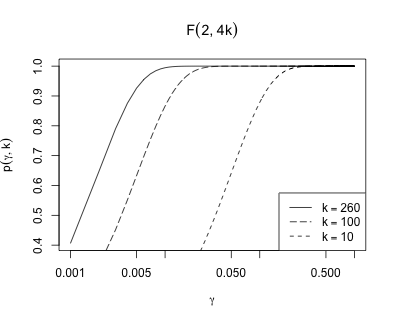The standard error in the admittance follows from the coherence spectrum: $\sqrt{(1 - \gamma^2)/k}$

G.err <- sqrt((1 - Coh) / k)


We can safely assume that the spectral density estimates for periods longer than $$\approx 100$$ seconds will be either spurious, or lacking in seismic energy, so we will exclude them.

csd <- data.frame(f, p, lf, lp, Coh, G, G.err, Phi = Phi * 180 / pi)
csd.f <- subset(csd, p <= 100)
is.sig <- csd.f\$Coh > coh.99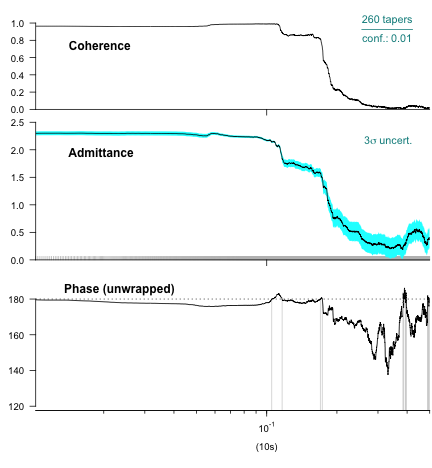This is now implemented in the function cross_spectrum. In comparison with a Welch-type CSD – calculated by setting k=NULL, the sine multitaper result is more accurate across the full frequency band, and does not degrade at low frequencies:

TohCS <- cross_spectrum(toh.dat, k=50, verbose=FALSE)
TohCS_welch <- cross_spectrum(toh.dat, k=NULL, verbose=FALSE) # turn off k to get a Welch overlapping csd
plot(Admittance ~ Period, TohCS, col=NA, log='x', main="Pore Pressure from Strain: Tohoku", xlab="Period, sec")
lines(Admittance ~ Period, TohCS_welch, col='salmon')
lines(Admittance ~ Period, TohCS, lwd=2)